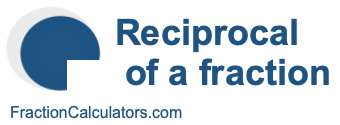Reciprocal of 1/3What is the reciprocal of 1/3? Here we will define reciprocal of 1/3, and show you how to calculate the reciprocal of 1/3 in fraction form and decimal form.

The reciprocal of 1/3 is a fraction or a number that when multiplied by 1/3 is equal to 1. To get the reciprocal of 1/3 based on that definition, we can make the following equation where "R" is the reciprocal of 1/3.

(1/3) × R = 1

When we solve for R, we get the answer to the reciprocal of 1/3 as a fraction as follows:

(1/3) × R = 1
R = 3/1
Reciprocal of 1/3 = 3/1

You can check that the answer is correct by confirming that 1/3 times the reciprocal of 1/3 is equal to 1, like this:

1/3 × 3/1 = 1

To find the decimal answer to the reciprocal of 1/3, you divide the numerator of the reciprocal by the demoninator of the reciprocal, like this:

3 ÷ 1 = 3
Reciprocal of 1/3 = 3

Tip: As you may have inferred from our tutorial above, you can quickly get the reciprocal of any fraction, such as 1/3, by switching the numerator and the denominator.

Reciprocal of a fraction
Do you need the reciprocal of another fraction? No problem! Please enter another fraction below.

Reciprocal of /

Reciprocal of 1/4
Here is the next fraction on our list that we have calculated the reciprocal of.